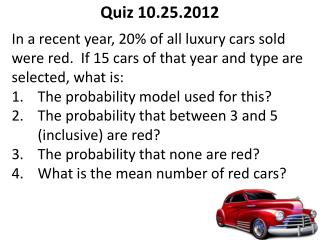DownloadDownload PresentationQuiz 10.25.2012

# Quiz 10.25.2012

Télécharger la présentation## Quiz 10.25.2012

- - - - - - - - - - - - - - - - - - - - - - - - - - - E N D - - - - - - - - - - - - - - - - - - - - - - - - - - -
##### Presentation Transcript

1. Quiz 10.25.2012 In a recent year, 20% of all luxury cars sold were red. If 15 cars of that year and type are selected, what is: The probability model used for this? The probability that between 3 and 5 (inclusive) are red? The probability that none are red? What is the mean number of red cars?

2. Quiz 10.25.2012 In a recent year, 20% of all luxury cars sold were red. If 15 cars of that year and type are selected, what is: Binomial with n = 15 and p = 0.20 P(X=3) + P(X=4) + P(X=5) = 0.2501 + 0.1876 + 0.1032 = 0.5409 P(X=0) = 0.03518 Mu = n*p = 15*0.20 = 3 Note: 2 and 3 can be solved easily with the Probability Distributions spreadsheet. Please see me if you do not understand how to use the spreadsheet or find the probability in minitab.